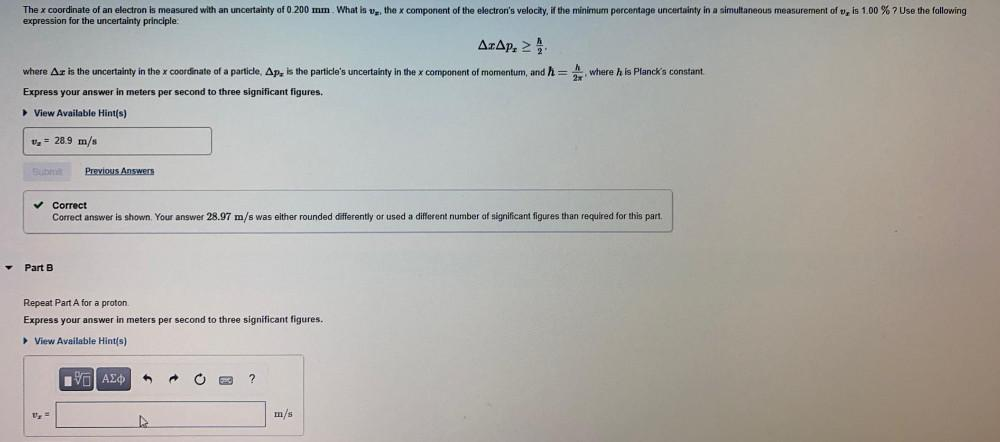Question:

# The x coordinate of an electron is measured with an uncertainty of 0.200 mm. What is Vz, the x component of the electron's velocThe x coordinate of an electron is measured with an uncertainty of 0.200 mm. What is Vz, the x component of the electron's velocity, if the minimum percentage uncertainty in a simultaneous measurement of v, is 1.00 % ? Use the following expression for the uncertainty principle: AcAp. where h is Planck's constant. where Az is the uncertainty in the x coordinate of a particle, Apz is the particle's uncertainty in the x component of momentum, and ħ= Express your answer in meters per second to three significant figures. View Available Hint(s) v2 = 28.9 m/s Sub Previous Answers Correct Correct answer is shown. Your answer 28.97 m/s was either rounded differently or used a different number of significant figures than required for this part. Part B Repeat Part A for a proton. Express your answer in meters per second to three significant figures. View Available Hint(s) IVO AEO ? Uz = m/s